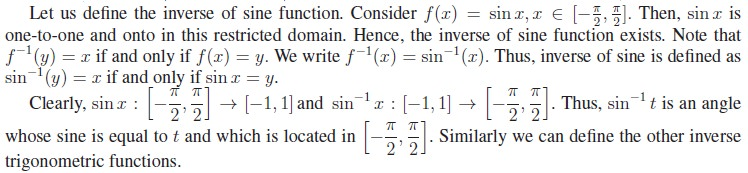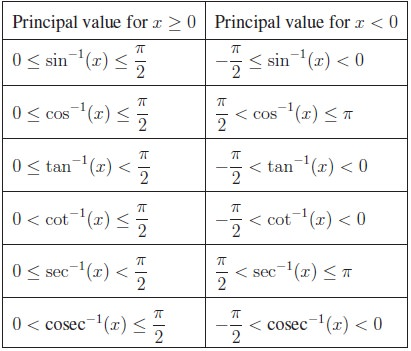Home | | Maths 11th std | Inverse Trigonometric Functions

# Inverse Trigonometric Functions

A function f(x) has inverse if and only if it is one-to-one and onto. Thus, inverse of a function cannot be defined if it fails to be one-to-one.

Inverse Trigonometric Functions

A function f(x) has inverse if and only if it is one-to-one and onto. Thus, inverse of a function cannot be defined if it fails to be one-to-one. However, if we restrict the domain suitably, we can make the function to be one-to-one in the restricted domain. For example, y = x2 is not one-to-one for all real numbers. But y = x2 is one-to-one and onto either for x Ōēź 0 or x Ōēż 0. Hence y = x2, x Ōēź 0 has the inverse f ŌłÆ1(x) = ŌłÜx, x Ōēź 0. Now, owing to their periodicity, none of six trigonometric functions is one-to-one over their natural domains. We shall restrict their domains so that trigonometric functions are one-to-one enabling the existence of their inverse functions. This restriction can be done in many ways once again due to their periodicity. The conventional choices for the restricted domains are arbitrary but they have some important characteristics. Each restricted domain includes the number 0 and some positive angles and the image of restricted domain contains the entire range.Tags : Definition, Formula, Solved Example Problems, Exercise | Mathematics , 11th Mathematics : UNIT 3 : Trigonometry
Study Material, Lecturing Notes, Assignment, Reference, Wiki description explanation, brief detail
11th Mathematics : UNIT 3 : Trigonometry : Inverse Trigonometric Functions | Definition, Formula, Solved Example Problems, Exercise | Mathematics